# 汉诺塔问题学习报告

## 一.基本汉诺塔的最少移动步数求解

• （如解析和参考有错误或可以精进之处，欢迎批评指正。）

（来源 百度百科）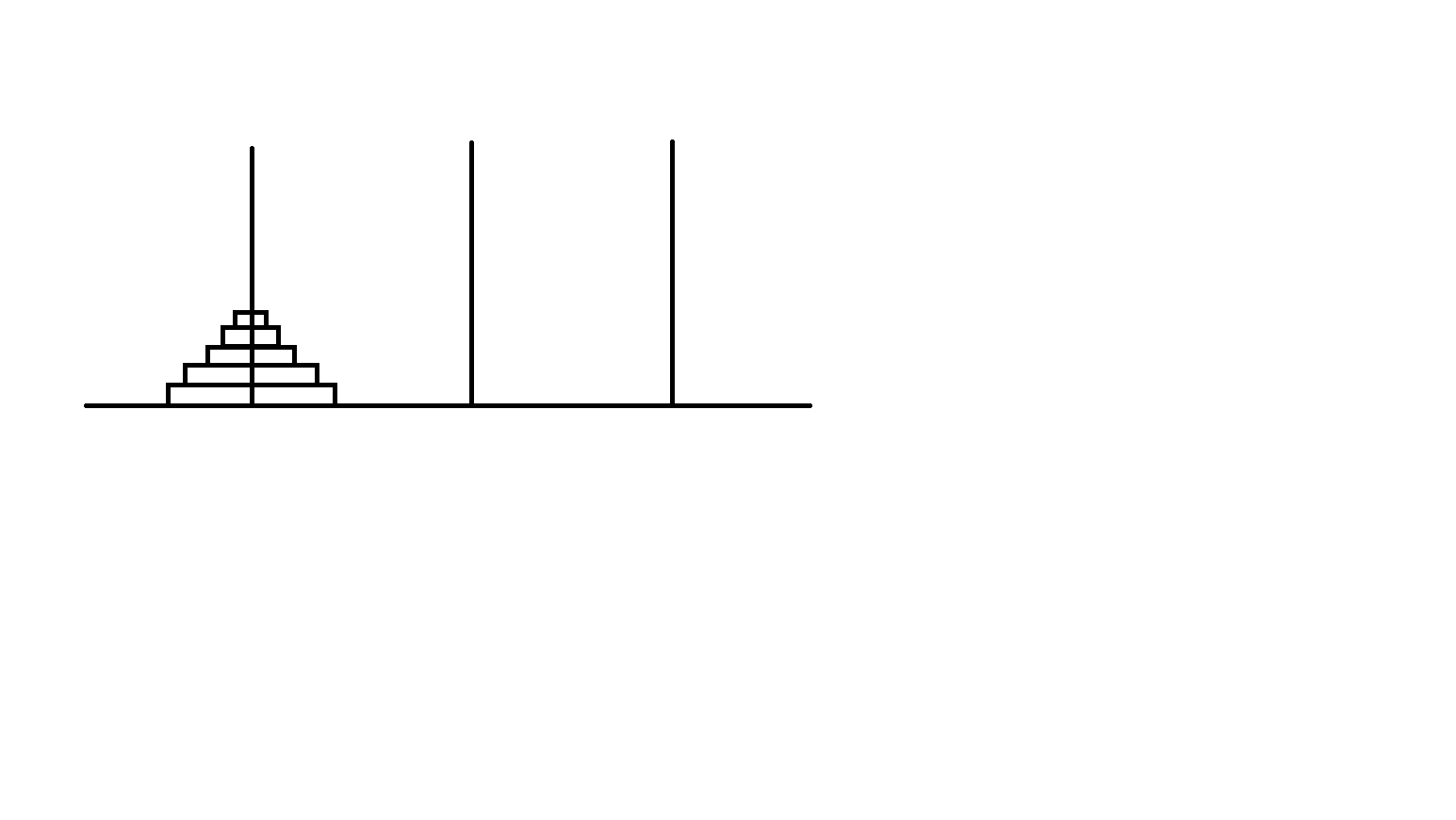例题1：现在有一个N层的汉诺塔，要解决这个汉诺塔问题，至少要移动多少步？ （难度1）

int a,n;
scanf("%d",&n);
a = 0;
for(i = 1;i <= n;i++){
a[i] = 2 * a[i - 1] + 1;
}
printf("%d",a[n]);


（注意：递推法不要忘记给起始条件）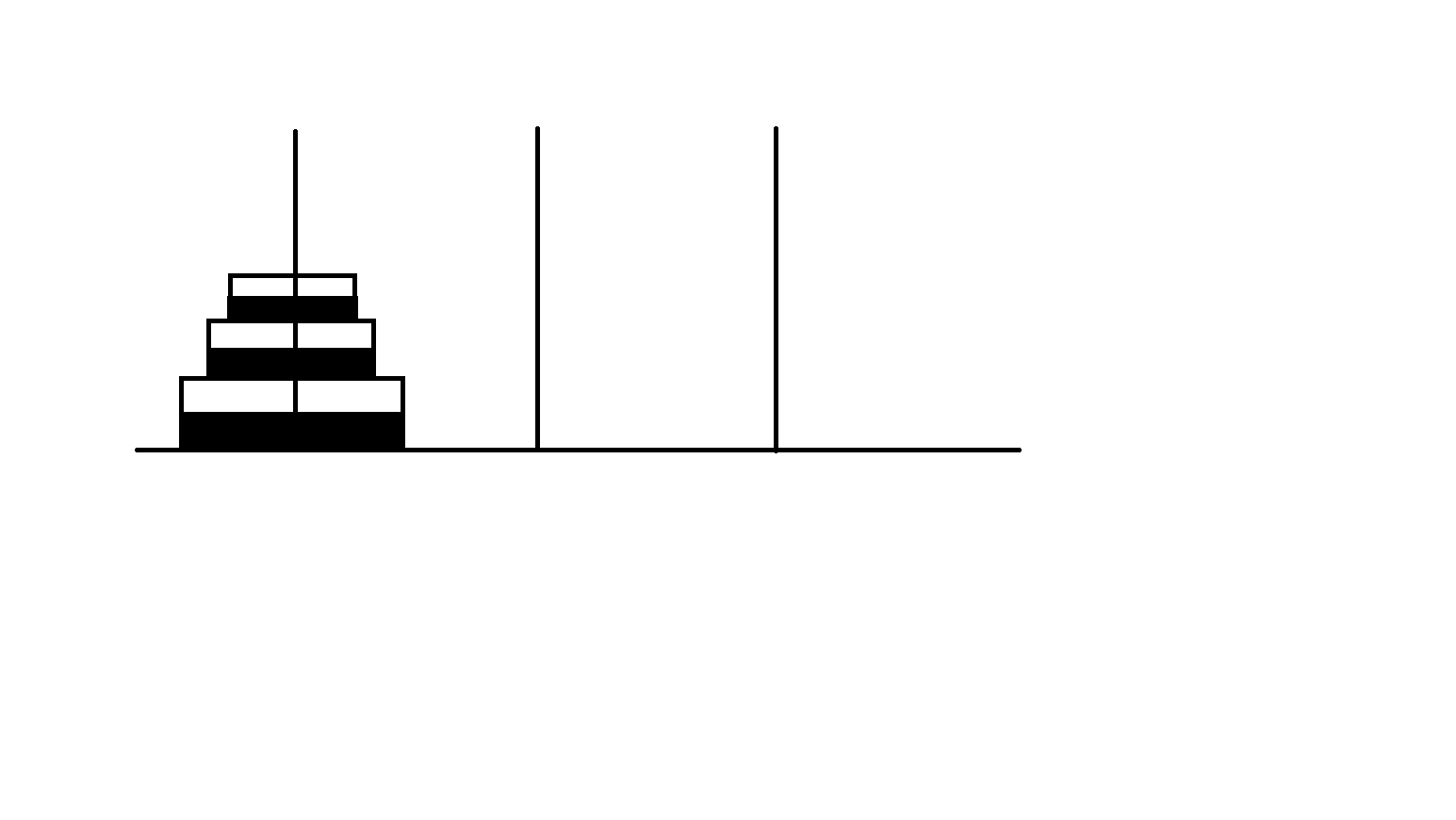## 二.汉诺塔操作的模拟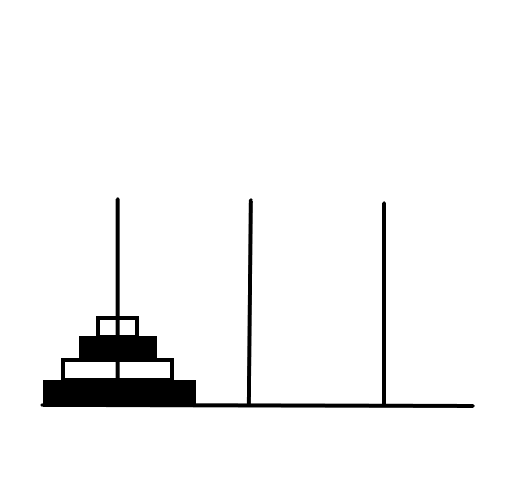int count;
void Move(int n, char a, char b){
count++;
printf("%d %c %c\n",n,a,b);
}
void Hanoi(int n, char a,char b,char c){//注解
if(n == 1) Move(n,a,c);
else{
Hanoi(n - 1,a,c,b);
Move(n,a,c);
Hanoi(n - 1,b,a,c);
}
}
int main(){
int n;
scanf("%d",&n);
Hanoi(n,'A','B','C');
printf("%d",count);
return 0;
}


（注：a相当于起点，b相当于终点，c相当于中转）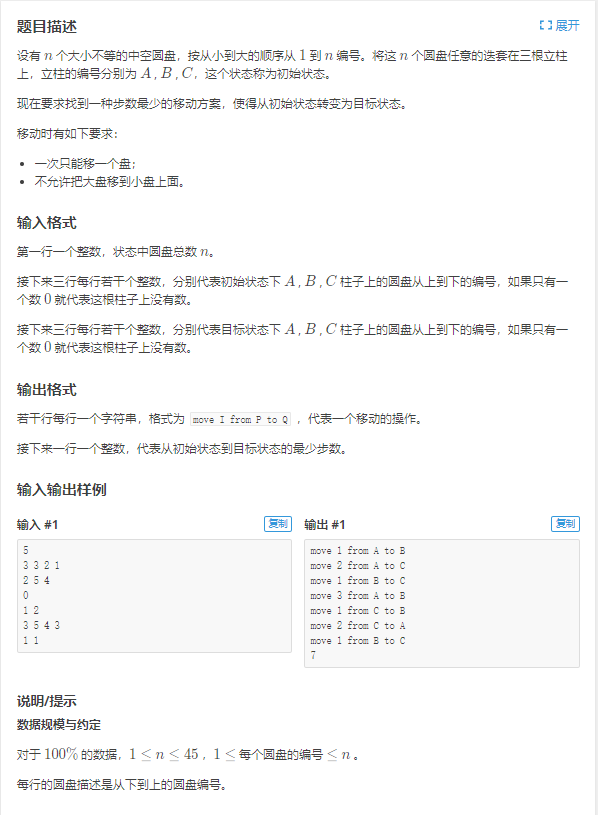struct yjx{
int from,to;
}a;//记每一层所在位置为from，目的地为to
int n,sum;
void hanoi(int x,int y){//把x移动到y位置去
if(a[x].from == y) return;//已到达的不必再排
else{
int i;
for(i = x - 1;i >= 1;i--) hanoi(i,6 - (a[x].from + y));//注解1
printf("move %d from %c to %c\n",x,a[x].from - 1 + 'A',y - 1 + 'A');//注解2
a[x].from = y;
sum++;
}
}
int main(){
......//输入部分略过
for(i = n;i >= 1;i--) hanoi(i,a[i].to);
printf("%d",sum);
return 0;
}//此题很容易混淆x和i、a[x].from和x，要多加注意


（注1：这里的6-(a[x].from + y)其实求的是某个盘的当前位置和目的地以外的那个柱，因为三个柱的和是6）
（注2：这里的一番操作是要把1、2、3转化为A、B、C）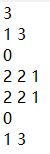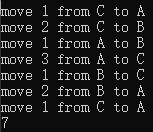（注意：以下完全属于本人分析，因此可能存在大量极其复杂反人类的内容，难以接受不建议阅读）

void hanoi(int x,int y){
if(a[x].from == y) return;
else{
int i;
lipu = 0,telipu = 1;
for(i = n;i >= 1;i--){
if(a[i].from == 6 - (a[x].from + y) && i != x && a[i].from != a[i].to || a[i].from == a[x].from && i < x || a[i].to == 6 - (a[x].from + y) && a[x].to != a[x].from){
lipu = 1;//前两条
break;
}
}
if(lipu == 0){
for(i = n;i >= 1;i--){
if(a[x].from == a[i].to && x != i && a[i].from != a[i].to){
telipu = 0;//第三条
break;
}
}
}
......//特判条件：lipu和telipu都为0


struct yjx{
int from,to;
}a;
int n,sum,ans;
bool go;
void hanoi(int w,int x,int y){//注解1
if(a[w][x].from == y) return;
else{
int i;
for(i = x - 1;i >= 1;i--){
if(a[w][i].from != 6 - (a[w][x].from + y)) hanoi(w,i,6 - (a[w][x].from + y));
}
if(go == 1) printf("move %d from %c to %c\n",x,a[w][x].from - 1 + 'A',y - 1 + 'A');
a[w][x].from = y;
sum++;
}
}
int main(){
for(i = n;i >= 1;i--) hanoi(1,i,a[i].to);
ans = sum;
sum = 0;
for(i = n;i >= 1;i--){
if(a[i].from != a[i].to){
hanoi(2,i,6 - (a[i].from + a[i].to));
break;//只需要操作一次
}
}
for(i = n;i >= 1;i--) hanoi(2,i,a[i].to);
ans = sum;
go = 1;
if(ans <= ans){
for(i = n;i >= 1;i--) hanoi(0,i,a[i].to);
}
if(ans > ans){
for(i = n;i >= 1;i--){
if(a[i].from != a[i].to){
hanoi(0,i,6 - (a[i].from + a[i].to));
break;
}
}
for(i = n;i >= 1;i--) hanoi(0,i,a[i].to);
}
printf("%d",min(ans,ans));
return 0;
}


（注1：这里多了一个w，是用来记录代表的是方法1，方法2还是答案。因为我们要操作三次，每次都是从初始状态开始操作，因此需要一个二维数组，省去初始化的麻烦）

## 三.汉诺塔变形问题

（难度3.5）

res[i] = 2 * res[j] + res1[i - j]

1 移动规则有优先度区分；
2 刚刚移动过的不能再移动。

#include<cstdio>
#include<cstring>
#include<algorithm>
using namespace std;
long long res;
void pre(){
int i,j;
for(i = 1;i <= 3;i++){
res[i] = 1;
if(i == 1){
for(j = 2;j <= 40;j++) res[i][j] = res[i][j - 1] * 2 + 1;
}
if(i == 2){
for(j = 2;j <= 40;j++) res[i][j] = res[i][j - 1] * 3 + 2;
}
if(i == 3){
for(j = 2;j <= 40;j++) res[i][j] = res[i - 1][j - 1] + res[i][j - 1] + 1;
}
}
}
int s1;
int main(){
int n,i,op,temp1,temp2;
char s;
scanf("%d",&n);
pre();
for(i = 1;i <= 6;i++){
scanf("%s",s[i]);
s1[s[i] - 'A' + 1][s[i] - 'A' + 1] = i;
}
//for(i = 1;i <= 3;i++) printf("%d/%d ",s1[i],s1[i]);
if(s1 < s1){//AB > AC
if(s1 < s1){//BA > BC
op = 2;
}
else{
if(s1 < s1){//CB > CA
op = 3;
}
else op = 1;
}
}
else{//AC > AB
if(s1 < s1){//CA > CB
op = 2;
}
else{
if(s1 < s1){//BC > BA
op = 3;
}
else op = 1;
}
}
printf("%lld\n",res[op][n]);
return 0;
}


12-29
11-27
05-15198
08-134537
11-10529
11-021032
09-053601
10-31225
06-161238
10-27313
09-192528
11-092658
01-26318
01-22119
02-241290
07-14725
10-03279
02-24725
11-273418
11-022254

### “相关推荐”对你有帮助么？

•非常没帮助
•没帮助
•一般
•有帮助
•非常有帮助被折叠的  条评论 为什么被折叠?到【灌水乐园】发言Hanoist

¥2 ¥4 ¥6 ¥10 ¥20余额支付 (余额：-- )扫码支付获取中扫码支付点击重新获取扫码支付1.余额是钱包充值的虚拟货币，按照1:1的比例进行支付金额的抵扣。
2.余额无法直接购买下载，可以购买VIP、C币套餐、付费专栏及课程。余额充值